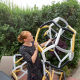# #MathArtChallenge Day 66: Venn DiagramsA complete 3 set Venn diagram, and an incomplete 4 set Venn diagram.

The challenge: Create a complete (all 16 spaces) 4 set venn diagram.

Materials needed: Pencil, paper, other??
Math concepts you could explore with this challenge: circles, combinations & permutations, graph theory, probability, vertices/intersections

Then, follow this to see some pretty spectacular math.

Depending on how you use this activity, you may engage with different mathematical standards. I’ve listed possible connected math content above. Here are a few suggestions for how you might integrate the 8 mathematical practices. Feel free to add your own suggestions in the comments!

2.) Reason abstractly and quantitatively. Calculate the number of spaces necessary to account for all of the possible combinations.

3.) Construct viable arguments and critique the reasoning of others. Once you have your image, create an argument to convince a skeptic that you have all necessary spaces.## Author: Ms. P

Math Teacher in Minneapolis, MN.

Posted on